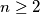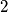### IMO Shortlist 1971 problem 6

Kvaliteta:
Avg: 0,0
Težina:
Avg: 0,0
Let$n \geq 2$ be a natural number. Find a way to assign natural numbers to the vertices of a regular$2n$-gon such that the following conditions are satisfied:

(1) only digits$1$ and$2$ are used;

(2) each number consists of exactly$n$ digits;

(3) different numbers are assigned to different vertices;

(4) the numbers assigned to two neighboring vertices differ at exactly one digit.
Izvor: Međunarodna matematička olimpijada, shortlist 1971